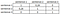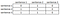# LexRank algorithm explained: a step-by-step tutorial with examplesImage by Nothing Ahead from Pexels

LexRank is a stochastic graph-based method for computing relative importance of textual units for Natural Language Processing. I used LexRank to summarize and extract the most relevant information from hundreds of articles, e.g. analysis on how to lose weight and how to succeed on a Medium as a writer. LexRank proved to work effectively — in my humble opinion.

At the first glance, the theory behind the algorithm seems complicated, but in reality, it’s very simple.

Let’s start with the official general description of the concept.

We consider a new approach, LexRank, for computing sentence importance based on the concept of eigenvector centrality in a graph representation of sentences. In this model, a connectivity matrix based on intra-sentence cosine similarity is used as the adjacency matrix of the graph representation of sentences.

Let’s divide LexRank algorithm into pieces so that step-by-step, with explained mathematical concepts, we get to the model output:

1. Input to the model
2. Word embeddings
3. Intra-sentence cosine similarity
4. Adjacency matrix
5. Connectivity matrix
6. Eigenvector centrality
7. Output of the model

The basic input to the model may be just an article or set of articles. It depends on whether your goal is to summarize one article or many articles.

LexRank can consume any text like:

• “This is an example sentence of the article. This is the second sentence. This is the third sentence, that is the most important cause it says other sentences are just examples.”

Most probably when we utilize LexRank in the above example it would give only the last sentence as a summary of the text.

Ok, but how to train a model with a text?

To apply any mathematical algorithm we need to convert the text input into numbers, typically in the form of a real-valued vectors. Representation of words in the vector format is called word embeddings.

Usually word embeddings are computed such that the words that are represented as similar vectors are expected to be similar in meaning.

Let’s see a simple example on cat, dog and aeroplane

`# example representation vector of a word "cat", "dog" and "aeroplane"cat_representation = [0, 0, 0, 1, 0, 1]dog_representation = [0, 0, 0, 1, 1, 1]aeroplane_represenatation = [1, 1, 0, 0, 0, 0]`

As you can see a “cat” and a “dog” representation in vectors are very similar whereas “aeroplane” is completely different from both animals words. In simple,“cat” and “dog” have common parts in the vector whereas aeroplane has zero common parts.

Note: generation of word embeddings is far beyond this tutorial. I will create another series of articles on it. To train your own word embeddings you would need a lot of data (like all articles from Wikipedia :)) and tremendous computing power.

Fortunately for us there are many already trained word embeddings that can be easily used e.g. from fast text here.

Ok, but why it is important? How can we use it?

We can use word embeddings in the sentence in many ways. However, in LexRank implementation an intra-sentence of sentence is used. It means the average of all word embeddings within a sentence that are used to compare to other sentences.

Let’s see an example

`sentence_1 = "this is a cat"sentence_2 = "this is a dog"# example representation of words in the form of vectors where each word is represented as a separate vectorthis_vector = [0,0,0]is_vector = [1,0,0]cat_vector = [0, 1, 0]dog_vector = [0, 0, 1]# how sentence looks in the form of vectors:sentence_1 = "[0,0,0] [1,0,0] [0, 1, 0]"sentence_2 = "[0,0,0] [1,0,0] [0, 0, 1]"# how to calculate intra sentence? Just take an averageintra_sentence_1 = [1/3, 1/3, 0]intra_sentence_2 = [1/3, 0, 1/3]`

But what’s a cosine similarity?

It’s also very easy! It’s just a metric (like any other), helpful in determining, how similar the data objects are irrespective of their size. In this case, how similar sentences are represented by vectors.

`The formula for calculating the cosine similarity is: Cos(x, y) = x . y / ||x|| * ||y||Where:x . y - a dot product || x || - length of a vector x|| y || - length of a vector y`

How to calculate it?

`x = sentence_1y = sentence_2x . y = 1/3*1/3 + 1/3*0 + 0*1/3 = 1/9|| x || = √ (1/3)^2 + (1/3)^2 + (0)^2 = 0.47|| y || = √ (1/3)^2 + (0)^2 + (1/3)^2 = 0.47Cos(x, y) = 1/9 / ((0.47) * (0.47)) = 0.024`

Easy, isn’t it?

And now the algorithm calculates it to every single sentence within a given text input.

But how can we represent it?

To represent a similarities across all sentences LexRank uses a connectivity matrix. Let’s see an example:

`# Example of adjacency matrix with weights as similaritiesLet's use previous sentences and add a new one with example similaritiessentence_1 = "this is a cat"sentence_2 = "this is a dog"sentence_3 = "this is an aeroplane"`example of adjacency matrix with similarities

As you can see sentence_1 and sentence_2 are similar whereas sentence_3 has no similarity with any other sentence.

There is one very big problem here. When you have many articles it might happen that in one article there will be many similar sentences. In such a case the model could output only sentences from this one article. In more technical terms it is called a local trap where few unimportant sentences votes to each other.

To get rid of the above problem LexRank takes into account that the importance of sentences should also stem from the importance of the sentences “recommending” it.

But how to do it?

Note: An adjacency matrix is usually a binary matrix with just information whether the two vertices have an edge between them. A connectivity matrix is usually a list of which vertex numbers have an edge between them.

To get rid of the local trap problem LexRank adds a count of connections from other sentences. In simple words, a sentence may have just 2 connections, but very strong, and win.

For example:

`sentence_1 is similar to 10 other sentencessentence_2 is similar to 6 other sentencessentence_3 is similar to sentence_1 and sentence_2sentence_3 is a winner because all connections from sentence_1 and setence_2 counts.`

To count the number of connection LexRank applies a threshold. For example, it only counts sentences as similar to itself where cosine similarity is more than 0.3 or 0.1.

Let’s see how the example connectivity matrix looks like with threshold 0.2:example connectivity matrix

As you can see sentence_3 is of the highest importance.

Last step — how to find all sentences with the highest score on the whole matrix?

To find out the most important sentences LexRank utilizes eigenvector centrality. The method is called power iteration method.

How it works?

• In the first step each matrix row is multiplied by a 1
• Secondly, we square rows results and take a root from the sum like: (1² + 1² + 2²)^(1/2)
• We repeat this step until the normalized value does not change much between any iteration

Finally, we have the output. In the above example sentence_3 got the highest score (0,667).

Hope it helps! Cheers!